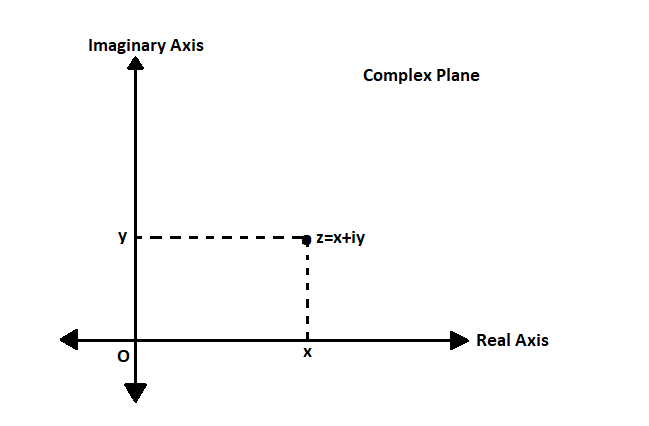What is the magnitude of the complex number 3 – 2i?

• Last Updated : 30 Nov, 2021

In mathematics, a number of the form x + iy where x, y ∈ R (set of real numbers) & i = √(-1) (also called iota) is called a complex number. Usually denoted as a complex number by z  i.e. z = x + iy where x represents the real part of the complex number z denoted by Re(z) and y represents the imaginary part of complex number z denoted by Im(z).

Representation of z=x+iy on Complex Plane

Complex Plane usually have cartesian co-ordinate system where the x-axis is the Real Axis representing the real part of the complex number and the y-axis is the Imaginary Axis representing the imaginary part of the complex number to study the geometric interpretation of complex numbers.Representation of z = x + iy on Complex Plane

Magnitude of a Complex Number

If z = x + iy is a complex number or a point in a complex plane, then the magnitude of a complex number z = x + iy denoted by |z| is the distance of the point z(x, y) from origin O(0, 0) in the complex plane. Magnitude is defined of a complex number z=x+iy as |z| = √(x2 + y2). Since distance is a scalar quantity, |z| ≥ 0 i.e. non-negative. Note that,

• Re(z) ≤ |Re(z)| ≤ |z|
• Im(z) ≤ |Im(z)| ≤ |z|

All the complex numbers having the same magnitude will lie on a circle having a center at the origin & radius r = |z|. Some important properties of the magnitude of complex numbers. If z1 and z2 are two complex numbers, then

• Magnitude over multiplication ⇢ |z1 × z2| = |z1| × |z2|
• Magnitude over division ⇢ |z1/z2| = |z1|/|z2| for z2 ≠ 0
• Triangle Inequality ⇢ |z1 + z2| ≤ |z1| + |z2
• Law of Parallelogram ⇢ |z1 + z2|2 + |z1 – z2|2 = 2 × {|z1|2 + |z2|2

Representation of |z| on Complex Plane,Representation of |z| on Complex Plane

What is the magnitude of the complex number 3-2i

Solution:

For complex number z = 3 – 2i,

The magnitude will be |z| = √(x2 + y2)

= √(32 + (-2)2

= √13

Similar Problems

Question 1: What is the magnitude of the complex number 5 + 3i?

Solution:

For complex number z = 5 + 3i,

The magnitude will be |z| = √(x2 + y2)

= √(52 + 32) = √34

Question 2: What is the magnitude of the complex number -2 + i?

Solution:

For complex number z = -2 + i,

The magnitude will be |z| = √(x2+y2)

= √((-2)2+12

= √5

Question 3: What is the magnitude of the complex number -5 + 2i?

Solution:

For complex number z = -5 + 2i,

The magnitude will be |z| = √(x2+y2)

= √((-5)2+22

= √29

My Personal Notes arrow_drop_up